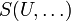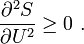# Le Chatelier's principle

This translates to conditions on the second derivatives of thermodynamic potentials such as entropy,$S(U,\ldots)$. For instance, the entropy is a concave function of its arguments such as internal energy. Thus, one has$\frac{\partial^2 S}{\partial U^2} \geq0\ .$